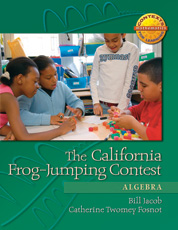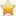# The California Frog-Jumping Contest

## Algebra

By William Jacob, Catherine Twomey Fosnot

The California Frog-Jumping Contest: Algebra is one of five units in the Contexts for Learning Mathematics’ Investigating Fractions, Decimals, and Percents (4–6)

This unit uses the context of the famous short story by Mark Twain—The Celebrated Jumping Frog of Calaveras County—to develop equivalence and its use in solving algebraic problems. The context of a frog jumping along a track is used to foster number line representations in which students solve for an unknown amount, which is usually the length of a frog jump. Equivalent sequences of jumps
...Special Offer: Save 30% off our list price automatically when you buy 15 or more.

### Paperback

In Stock

List Price: \$31.25

Web/School Price: \$25.00

##### PART OF THE SERIES

The Context for Learning Mathematics Series

#### Full Description

The California Frog-Jumping Contest: Algebra is one of five units in the Contexts for Learning Mathematics’ Investigating Fractions, Decimals, and Percents (4–6)

This unit uses the context of the famous short story by Mark Twain—The Celebrated Jumping Frog of Calaveras County—to develop equivalence and its use in solving algebraic problems. The context of a frog jumping along a track is used to foster number line representations in which students solve for an unknown amount, which is usually the length of a frog jump. Equivalent sequences of jumps are represented naturally on a double number line by having them start and end at the same location, with one expression shown on top of the line and the other shown underneath the line. The representation can then be used as a tool for solving the problem.

The unit begins with a problem in which students find the length of a bullfrog’s jump, knowing the full length of a sequence of his jumps and steps. This context leads to using the number line as a tool for solving problems with unknowns. Next, students must find various approaches for lining up six- or eight-foot benches for two jumping tracks of lengths 28 and 42 feet. Students utilize the equivalence 6 + 6 + 6 + 6 = 8 + 8 + 8 to change one possible solution into a second possible solution and use the number line to represent this equivalence. A similar problem about fences is used to develop a combination chart, which is a useful representation for determining net gain (or loss) after an exchange.

The second half of the unit includes more frog-jumping problems as the frogs plan for their Olympic Games. Now students further explore the use of variables to represent more complex situations and solve for unknown amounts. Here, students use the number line to represent jumps in the problems and can separate off equal amounts of unknown lengths to determine the lengths of unknown amounts. As the unit progresses, the questions require that students investigate equivalent lengths of different-sized jumps and work with these equivalences flexibly to solve problems.

The complexity of learning to symbolize has been the subject of extensive research. One study, summarized in Adding It Up (National Research Council 2001, 264), illustrates typical difficulties students may have. Known as the reversal error, it is illustrated by work on the following problem: At a certain university, there are six times as many students as professors. Using S for the number of students and P for the number of professors, write an equation that gives the relation between the number of students and the number of professors. A majority of students, ranging from first-year algebra students to college freshmen, wrote the equation 6S=P. Apparently they used 6 as an adjective and S as a noun, following the natural language in the problem. However, they needed to multiply the number of professors by 6 to find the number of students. The correct response is 6P=S. Because learning to write algebraic expressions is so difficult, we don’t push symbolizing early in this unit. The representation of the number line is used to fix students’ attention on the distinction between the lengths of jumps and the number of jumps. Once this is set, students can begin symbolizing in problems like this in a meaningful way. The unit ends with the students constructing more formal algebraic notation as they develop methods to simplify their earlier representations.